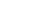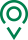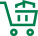• EN
• 圣康首页
• {if condition="\$categorys[\$vo]['is_menu'] == 1"}
• 关于圣康
• {if condition="\$categorys[\$vo]['is_menu'] == 1"} {if condition="\$categorys[\$vo]['is_menu'] == 1"}
• 产品展示
• {if condition="\$categorys[\$vo]['is_menu'] == 1"} {if condition="\$categorys[\$vo]['is_menu'] == 1"}
• 荣誉资质
• {if condition="\$categorys[\$vo]['is_menu'] == 1"} {if condition="\$categorys[\$vo]['is_menu'] == 1"}
• 新闻资讯
• {if condition="\$categorys[\$vo]['is_menu'] == 1"} {if condition="\$categorys[\$vo]['is_menu'] == 1"}
• 联系我们
• {if condition="\$categorys[\$vo]['is_menu'] == 1"} {if condition="\$categorys[\$vo]['is_menu'] == 1"}
• 在线留言
• {if condition="\$categorys[\$vo]['is_menu'] == 1"} {if condition="\$categorys[\$vo]['is_menu'] == 1"}
• 质量管控
• {if condition="\$categorys[\$vo]['is_menu'] == 1"} {if condition="\$categorys[\$vo]['is_menu'] == 1"}
• 源头种植
• {if condition="\$categorys[\$vo]['is_menu'] == 1"} {if condition="\$categorys[\$vo]['is_menu'] == 1"}
• 过程控制
• {if condition="\$categorys[\$vo]['is_menu'] == 1"}

# 食安检测技术示范中心公示 质谱仪、色谱仪打头阵

11月12日，工业和信息化部消费品工业司对“食品企业质量安全检测技术示范中心”名单(第五批)进行公示。当前赞宇科技集团股份有限公司(浙江省轻工业研究所)、福建赛福食品检测研究所有限公司、湖南省食品测试分析中心、西安国联质量检测技术股份有限公司四家企业位于名单上。建立“食品企业质量安全检测技术示范中心”意味着要为中小食品生产企业提供检测示范、人员培训、技术咨询、委托检测和应急检测服务等方面的服务，需要相关企业自身具备强大的食品质量安全检测能力。

赞宇科技集团股份有限公司

赞宇科技集团股份有限公司前身是浙江省轻工业研究所，是一家能够提供食品安全、环境、职业卫生等第三方检测认证服务的企业。当前，赞宇科技共拥有气-质联用仪、液相色谱质谱仪、电感耦合等离子体质谱仪、离子色谱仪、气相色谱仪、液相色谱仪、高效毛细管电泳仪、酶标仪等大型精密仪器百余台。

除了设备齐全，赞宇科技还不断深入食品检测，探求更高品质、更快速的食品检测服务。基于三氧化硫气相膜式磺化技术，企业相关人员研发出“大豆磷脂气相膜式磺化制取表面活性剂”，工艺技术属国内领先；还率先提出并实施以液体三氧化硫替代气相三氧化硫的磺化新工艺，这大大简化了流程，节省了投资，充分体现节能、环保、清洁生产和循环经济的设计理念。

### 我们的宗旨

•全球精选

一站式购物方案

•多仓直发

特有次日达配送服务

•源头直采

精选正宗好食材

•天天底价

新鲜到家购不停